# Regularization in deep learning

27. May 2019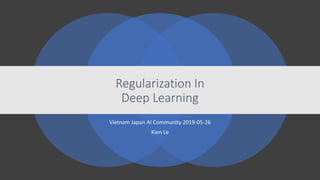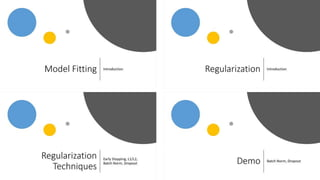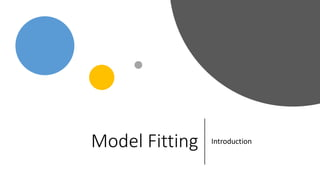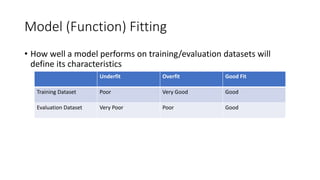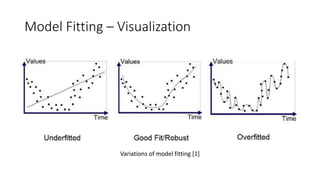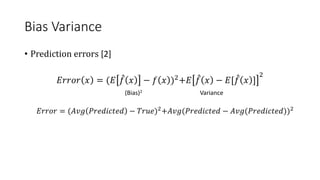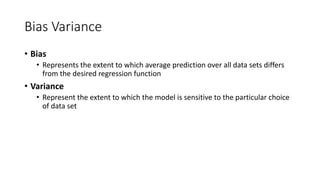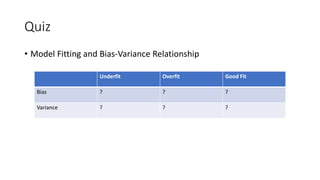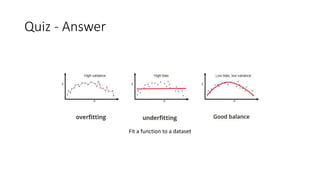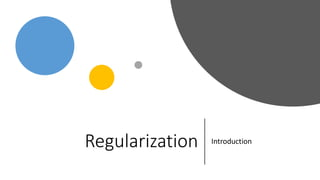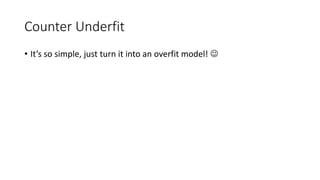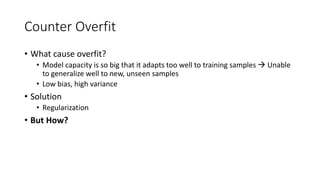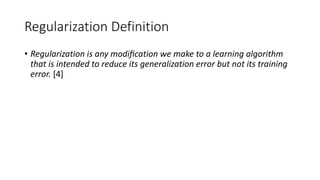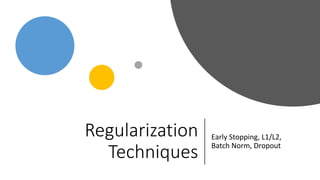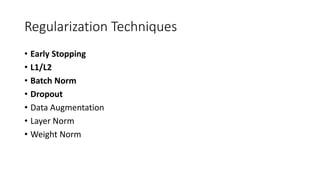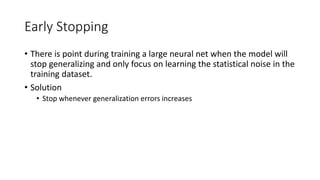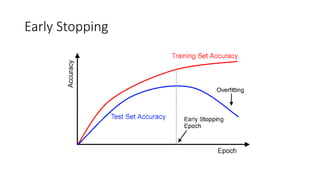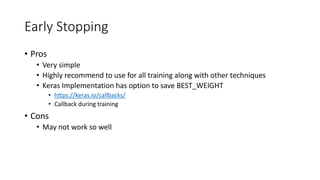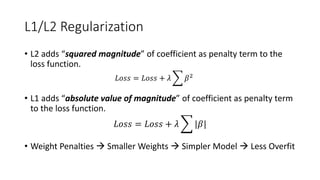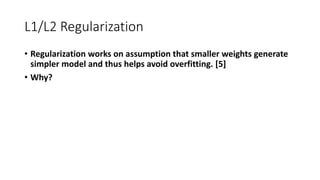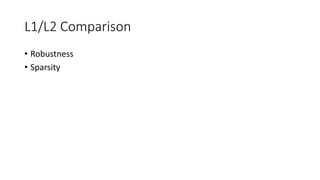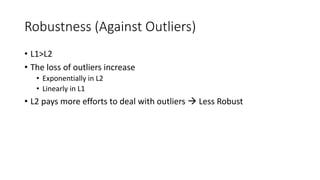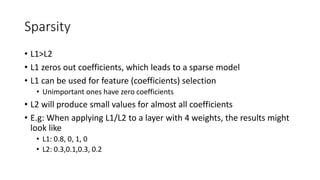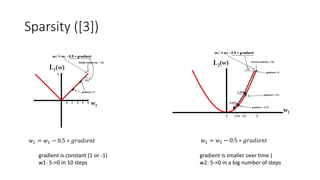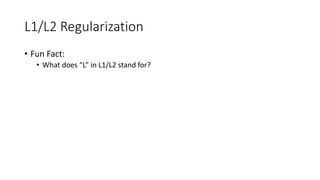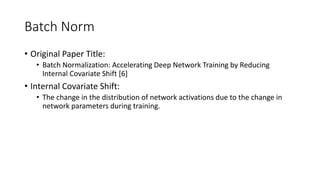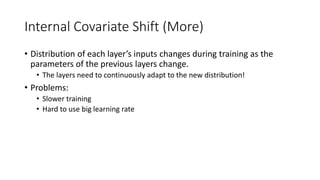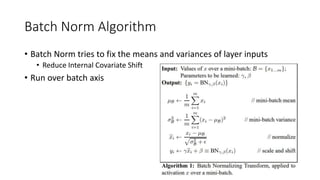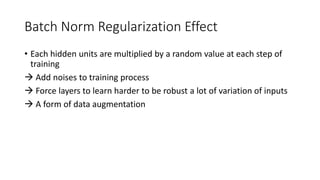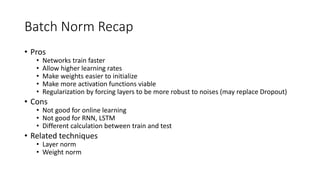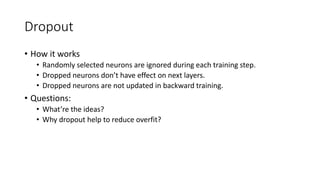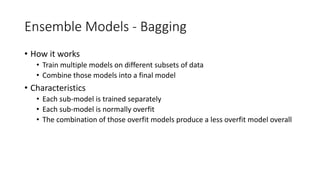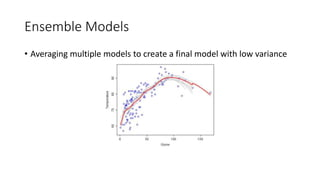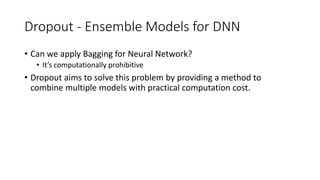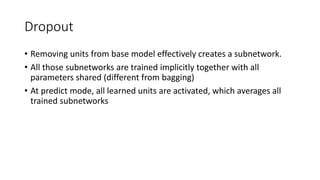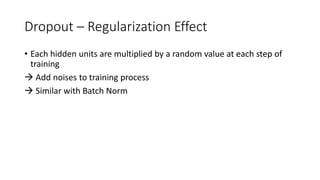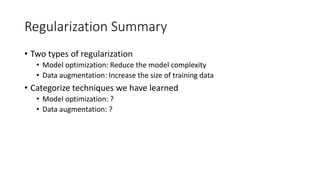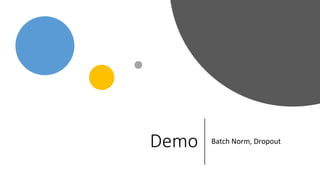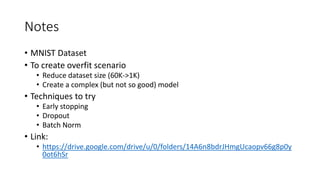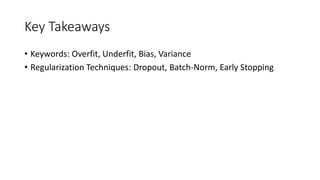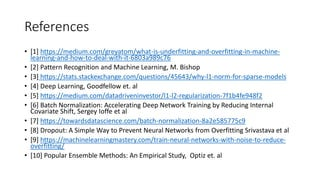1 von 42

### Regularization in deep learning

• 1. Vietnam Japan AI Community 2019-05-26 Kien Le Regularization In Deep Learning
• 4. Model (Function) Fitting • How well a model performs on training/evaluation datasets will define its characteristics Underfit Overfit Good Fit Training Dataset Poor Very Good Good Evaluation Dataset Very Poor Poor Good
• 5. Model Fitting – Visualization Variations of model fitting 
• 6. Bias Variance • Prediction errors  𝐸𝑟𝑟𝑜𝑟 𝑥 = (𝐸 𝑓 𝑥 − 𝑓 𝑥 )2+𝐸 𝑓 𝑥 − 𝐸[ 𝑓 𝑥 ] 2 (Bias)2 Variance 𝐸𝑟𝑟𝑜𝑟 = (𝐴𝑣𝑔 𝑃𝑟𝑒𝑑𝑖𝑐𝑡𝑒𝑑 − 𝑇𝑟𝑢𝑒)2+𝐴𝑣𝑔(𝑃𝑟𝑒𝑑𝑖𝑐𝑡𝑒𝑑 − 𝐴𝑣𝑔(𝑃𝑟𝑒𝑑𝑖𝑐𝑡𝑒𝑑))2
• 7. Bias Variance • Bias • Represents the extent to which average prediction over all data sets differs from the desired regression function • Variance • Represent the extent to which the model is sensitive to the particular choice of data set
• 8. Quiz • Model Fitting and Bias-Variance Relationship Underfit Overfit Good Fit Bias ? ? ? Variance ? ? ?
• 9. Quiz - Answer Fit a function to a dataset
• 11. Counter Underfit • What causes underfit? • Model capacity is too small to fit the training dataset as well as generalize to new dataset. • High bias, low variance • Solution • Increase the capacity of the model • Examples: • Increase number of layers, neurons in each layer, etc. • Result: • Lower Bias • Underfit  Good Fit?
• 12. Counter Underfit • It’s so simple, just turn it into an overfit model! 
• 13. Counter Overfit • What cause overfit? • Model capacity is so big that it adapts too well to training samples  Unable to generalize well to new, unseen samples • Low bias, high variance • Solution • Regularization • But How?
• 14. Regularization Definition • Regularization is any modiﬁcation we make to a learning algorithm that is intended to reduce its generalization error but not its training error. 
• 16. Regularization Techniques • Early Stopping • L1/L2 • Batch Norm • Dropout • Data Augmentation • Layer Norm • Weight Norm
• 17. Early Stopping • There is point during training a large neural net when the model will stop generalizing and only focus on learning the statistical noise in the training dataset. • Solution • Stop whenever generalization errors increases
• 19. Early Stopping • Pros • Very simple • Highly recommend to use for all training along with other techniques • Keras Implementation has option to save BEST_WEIGHT • https://keras.io/callbacks/ • Callback during training • Cons • May not work so well
• 20. L1/L2 Regularization • L2 adds “squared magnitude” of coefficient as penalty term to the loss function. 𝐿𝑜𝑠𝑠 = 𝐿𝑜𝑠𝑠 + 𝜆 𝛽2 • L1 adds “absolute value of magnitude” of coefficient as penalty term to the loss function. 𝐿𝑜𝑠𝑠 = 𝐿𝑜𝑠𝑠 + 𝜆 |𝛽| • Weight Penalties  Smaller Weights  Simpler Model  Less Overfit
• 21. L1/L2 Regularization • Regularization works on assumption that smaller weights generate simpler model and thus helps avoid overfitting.  • Why?
• 23. Robustness (Against Outliers) • L1>L2 • The loss of outliers increase • Exponentially in L2 • Linearly in L1 • L2 pays more efforts to deal with outliers  Less Robust
• 24. Sparsity • L1>L2 • L1 zeros out coefficients, which leads to a sparse model • L1 can be used for feature (coefficients) selection • Unimportant ones have zero coefficients • L2 will produce small values for almost all coefficients • E.g: When applying L1/L2 to a layer with 4 weights, the results might look like • L1: 0.8, 0, 1, 0 • L2: 0.3,0.1,0.3, 0.2
• 25. Sparsity () 𝑤1 = 𝑤1 − 0.5 ∗ 𝑔𝑟𝑎𝑑𝑖𝑒𝑛𝑡 𝑤1 = 𝑤1 − 0.5 ∗ 𝑔𝑟𝑎𝑑𝑖𝑒𝑛𝑡 gradient is constant (1 or -1) w1: 5->0 in 10 steps gradient is smaller over time ( w2: 5->0 in a big number of steps
• 26. L1/L2 Regularization • Fun Fact: • What does “L” in L1/L2 stand for?
• 27. Batch Norm • Original Paper Title: • Batch Normalization: Accelerating Deep Network Training by Reducing Internal Covariate Shift  • Internal Covariate Shift: • The change in the distribution of network activations due to the change in network parameters during training.
• 28. Internal Covariate Shift (More) • Distribution of each layer’s inputs changes during training as the parameters of the previous layers change. • The layers need to continuously adapt to the new distribution! • Problems: • Slower training • Hard to use big learning rate
• 29. Batch Norm Algorithm • Batch Norm tries to fix the means and variances of layer inputs • Reduce Internal Covariate Shift • Run over batch axis
• 30. Batch Norm Regularization Effect • Each hidden units are multiplied by a random value at each step of training  Add noises to training process  Force layers to learn harder to be robust a lot of variation of inputs  A form of data augmentation
• 31. Batch Norm Recap • Pros • Networks train faster • Allow higher learning rates • Make weights easier to initialize • Make more activation functions viable • Regularization by forcing layers to be more robust to noises (may replace Dropout) • Cons • Not good for online learning • Not good for RNN, LSTM • Different calculation between train and test • Related techniques • Layer norm • Weight norm
• 32. Dropout • How it works • Randomly selected neurons are ignored during each training step. • Dropped neurons don’t have effect on next layers. • Dropped neurons are not updated in backward training. • Questions: • What’re the ideas? • Why dropout help to reduce overfit?
• 33. Ensemble Models - Bagging • How it works • Train multiple models on different subsets of data • Combine those models into a final model • Characteristics • Each sub-model is trained separately • Each sub-model is normally overfit • The combination of those overfit models produce a less overfit model overall
• 34. Ensemble Models • Averaging multiple models to create a final model with low variance
• 35. Dropout - Ensemble Models for DNN • Can we apply Bagging for Neural Network? • It’s computationally prohibitive • Dropout aims to solve this problem by providing a method to combine multiple models with practical computation cost.
• 36. Dropout • Removing units from base model effectively creates a subnetwork. • All those subnetworks are trained implicitly together with all parameters shared (different from bagging) • At predict mode, all learned units are activated, which averages all trained subnetworks
• 37. Dropout – Regularization Effect • Each hidden units are multiplied by a random value at each step of training  Add noises to training process  Similar with Batch Norm
• 38. Regularization Summary • Two types of regularization • Model optimization: Reduce the model complexity • Data augmentation: Increase the size of training data • Categorize techniques we have learned • Model optimization: ? • Data augmentation: ?
• 39. Demo Batch Norm, Dropout
• 40. Notes • MNIST Dataset • To create overfit scenario • Reduce dataset size (60K->1K) • Create a complex (but not so good) model • Techniques to try • Early stopping • Dropout • Batch Norm • Link: • https://drive.google.com/drive/u/0/folders/14A6n8bdrJHmgUcaopv66g8p0y 0ot6hSr
• 41. Key Takeaways • Keywords: Overfit, Underfit, Bias, Variance • Regularization Techniques: Dropout, Batch-Norm, Early Stopping
• 42. References •  https://medium.com/greyatom/what-is-underfitting-and-overfitting-in-machine- learning-and-how-to-deal-with-it-6803a989c76 •  Pattern Recognition and Machine Learning, M. Bishop •  https://stats.stackexchange.com/questions/45643/why-l1-norm-for-sparse-models •  Deep Learning, Goodfellow et. al •  https://medium.com/datadriveninvestor/l1-l2-regularization-7f1b4fe948f2 •  Batch Normalization: Accelerating Deep Network Training by Reducing Internal Covariate Shift, Sergey Ioffe et al •  https://towardsdatascience.com/batch-normalization-8a2e585775c9 •  Dropout: A Simple Way to Prevent Neural Networks from Overfitting Srivastava et al •  https://machinelearningmastery.com/train-neural-networks-with-noise-to-reduce- overfitting/ •  Popular Ensemble Methods: An Empirical Study, Optiz et. al

### Hinweis der Redaktion

1. There are two common sources of variance in a final model: The noise in the training data. The use of randomness in the machine learning algorithm.
2. Lebesgue